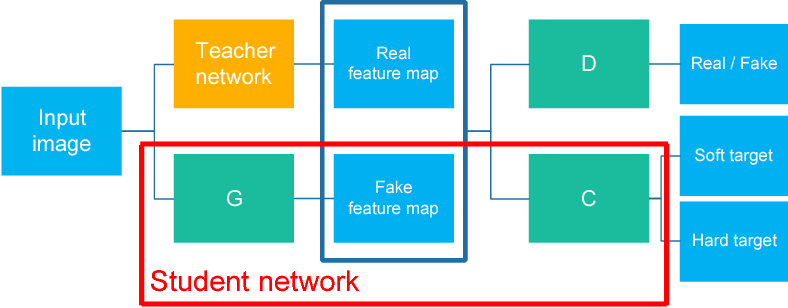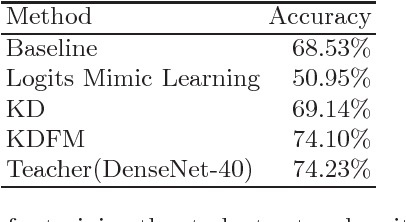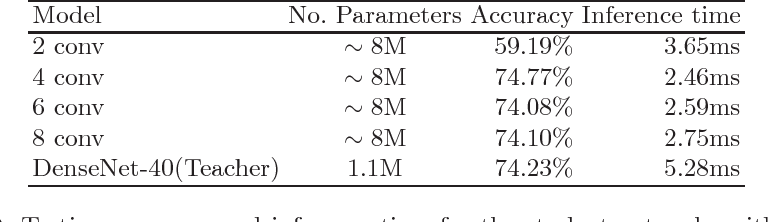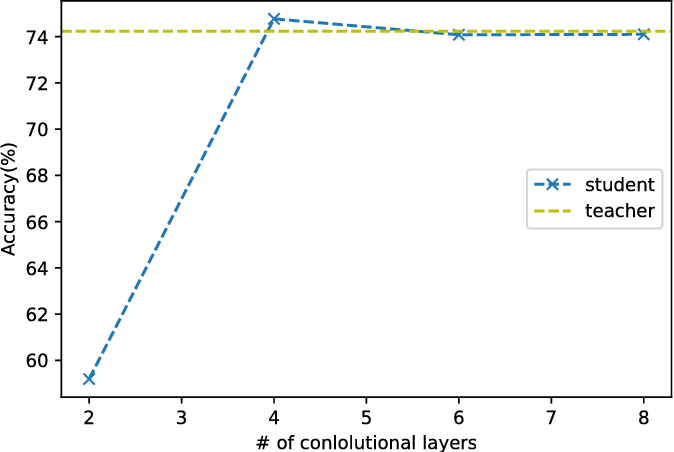Research papers and code for "Chien-Yu Chen":
In recent years, kernel density estimation has been exploited by computer scientists to model machine learning problems. The kernel density estimation based approaches are of interest due to the low time complexity of either O(n) or O(n*log(n)) for constructing a classifier, where n is the number of sampling instances. Concerning design of kernel density estimators, one essential issue is how fast the pointwise mean square error (MSE) and/or the integrated mean square error (IMSE) diminish as the number of sampling instances increases. In this article, it is shown that with the proposed kernel function it is feasible to make the pointwise MSE of the density estimator converge at O(n^-2/3) regardless of the dimension of the vector space, provided that the probability density function at the point of interest meets certain conditions.

* The new version includes an additional theorem, Theorem 3
Click to Read Paper and Get CodeThe model reduction problem that eases the computation costs and latency of complex deep learning architectures has received an increasing number of investigations owing to its importance in model deployment. One promising method is knowledge distillation (KD), which creates a fast-to-execute student model to mimic a large teacher network. In this paper, we propose a method, called KDFM (Knowledge Distillation with Feature Maps), which improves the effectiveness of KD by learning the feature maps from the teacher network. Two major techniques used in KDFM are shared classifier and generative adversarial network. Experimental results show that KDFM can use a four layers CNN to mimic DenseNet-40 and use MobileNet to mimic DenseNet-100. Both student networks have less than 1\% accuracy loss comparing to their teacher models for CIFAR-100 datasets. The student networks are 2-6 times faster than their teacher models for inference, and the model size of MobileNet is less than half of DenseNet-100's.

* Knowledge Distillation, Model Compression, and Generative Adversarial Network, ACCV 2018
Click to Read Paper and Get Code
This article proposes a novel density estimation based algorithm for carrying out supervised machine learning. The proposed algorithm features O(n) time complexity for generating a classifier, where n is the number of sampling instances in the training dataset. This feature is highly desirable in contemporary applications that involve large and still growing databases. In comparison with the kernel density estimation based approaches, the mathe-matical fundamental behind the proposed algorithm is not based on the assump-tion that the number of training instances approaches infinite. As a result, a classifier generated with the proposed algorithm may deliver higher prediction accuracy than the kernel density estimation based classifier in some cases.

* Inclusion of a new "Remarks" section
Click to Read Paper and Get Code